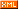# zero_learner

 博客园 :: 首页 :: 博问 :: 闪存 :: 新随笔 :: 联系 :: 订阅:: 管理 ::# GBDT算法原理深入解析

Boosting、bagging和stacking是集成学习的三种主要方法。不同于bagging方法，boosting方法通过分步迭代（stage-wise）的方式来构建模型，在迭代的每一步构建的弱学习器都是为了弥补已有模型的不足。Boosting族算法的著名代表是AdaBoost，AdaBoost算法通过给已有模型预测错误的样本更高的权重，使得先前的学习器做错的训练样本在后续受到更多的关注的方式来弥补已有模型的不足。与AdaBoost算法不同，梯度提升方法在迭代的每一步构建一个能够沿着梯度最陡的方向降低损失（steepest-descent）的学习器来弥补已有模型的不足。经典的AdaBoost算法只能处理采用指数损失函数的二分类学习任务，而梯度提升方法通过设置不同的可微损失函数可以处理各类学习任务（多分类、回归、Ranking等），应用范围大大扩展。另一方面，AdaBoost算法对异常点（outlier）比较敏感，而梯度提升算法通过引入bagging思想、加入正则项等方法能够有效地抵御训练数据中的噪音，具有更好的健壮性。这也是为什么梯度提升算法（尤其是采用决策树作为弱学习器的GBDT算法）如此流行的原因，有种观点认为GBDT是性能最好的机器学习算法，这当然有点过于激进又固步自封的味道，但通常各类机器学习算法比赛的赢家们都非常青睐GBDT算法，由此可见该算法的实力不可小觑。

## 加法模型（additive model）

GBDT算法可以看成是由K棵树组成的加法模型：$$\hat{y}i=\sum{k=1}^K f_k(x_i), f_k \in F \tag 0$$

$\begin{split} \hat{y}_i^0 &= 0 \\ \hat{y}_i^1 &= f_1(x_i) = \hat{y}_i^0 + f_1(x_i) \\ \hat{y}_i^2 &= f_1(x_i) + f_2(x_i) = \hat{y}_i^1 + f_2(x_i) \\ & \cdots \\ \hat{y}_i^t &= \sum_{k=1}^t f_k(x_i) = \hat{y}_i^{t-1} + f_t(x_i) \\ \end{split}$

$\begin{split} Obj^{(t)} &= \sum_{i=1}^nl(y_i, \hat{y}_i^t) + \sum_{i=i}^t \Omega(f_i) \\ &= \sum_{i=1}^n l\left(y_i, \hat{y}_i^{t-1} + f_t(x_i) \right) + \Omega(f_t) + constant \end{split}\tag{1}$

$\begin{split} Obj^{(t)} &= \sum_{i=1}^n \left(y_i - (\hat{y}_i^{t-1} + f_t(x_i)) \right)^2 + \Omega(f_t) + constant \\ &= \sum_{i=1}^n \left[2(\hat{y}_i^{t-1} - y_i)f_t(x_i) + f_t(x_i)^2 \right] + \Omega(f_t) + constant \end{split}\tag{2}$

----维基百科

$f(x+\Delta x) \approx f(x) + f'(x)\Delta x + \frac12 f''(x)\Delta x^2 \tag 3$

$Obj^{(t)} = \sum_{i=1}^n \left[ l(y_i, \hat{y}_i^{t-1}) + g_if_t(x_i) + \frac12h_if_t^2(x_i) \right] + \Omega(f_t) + constant \tag 4$

$Obj^{(t)} \approx \sum_{i=1}^n \left[ g_if_t(x_i) + \frac12h_if_t^2(x_i) \right] + \Omega(f_t) \tag 5$

## GBDT算法

$\begin{split} Obj^{(t)} &\approx \sum_{i=1}^n \left[ g_if_t(x_i) + \frac12h_if_t^2(x_i) \right] + \Omega(f_t) \\ &= \sum_{i=1}^n \left[ g_iw_{q(x_i)} + \frac12h_iw_{q(x_i)}^2 \right] + \gamma T + \frac12 \lambda \sum_{j=1}^T w_j^2 \\ &= \sum_{j=1}^T \left[(\sum_{i \in I_j}g_i)w_j + \frac12(\sum_{i \in I_j}h_i + \lambda)w_j^2 \right] + \gamma T \end{split}\tag 6$

$Obj^{(t)} = \sum_{j=1}^T \left[G_iw_j + \frac12(H_i + \lambda)w_j^2 \right] + \gamma T$

1. 枚举所有可能的树结构$q$
2. 用等式(8)为每个$q$计算其对应的分数$Obj$，分数越小说明对应的树结构越好
3. 根据上一步的结果，找到最佳的树结构，用等式(7)为树的每个叶子节点计算预测值

1. 从深度为0的树开始，对每个叶节点枚举所有的可用特征
2. 针对每个特征，把属于该节点的训练样本根据该特征值升序排列，通过线性扫描的方式来决定该特征的最佳分裂点，并记录该特征的最大收益（采用最佳分裂点时的收益）
3. 选择收益最大的特征作为分裂特征，用该特征的最佳分裂点作为分裂位置，把该节点生长出左右两个新的叶节点，并为每个新节点关联对应的样本集
4. 回到第1步，递归执行到满足特定条件为止

1. 算法每次迭代生成一颗新的决策树
2. 在每次迭代开始之前，计算损失函数在每个训练样本点的一阶导数$g_i$和二阶导数$h_i$
3. 通过贪心策略生成新的决策树，通过等式(7)计算每个叶节点对应的预测值
4. 把新生成的决策树$f_t(x)$添加到模型中：$\hat{y}_i^t = \hat{y}_i^{t-1} + f_t(x_i)$

## 参考资料

 Gradient Boosting 的更多内容
 XGBoost 是一个优秀的GBDT开源软件库，有多种语言接口
 Pyramid 是一个基于Java语言的机器学习库，里面也有GBDT算法的介绍和实现
 Friedman的论文《Greedy function approximation: a gradient boosting machine》是比较早的GBDT算法文献，但是比较晦涩难懂，不适合初学者，高阶选手可以进一步学习
 "A Gentle Introduction to Gradient Boosting"是关于Gradient Boosting的一个通俗易懂的解释，比较适合初学者或者是已经对GBDT算法原理印象不深的从业者
 关于GBDT算法调参的经验和技巧可以参考这两篇博文：《GBM调参指南》、
XGBoost调参指南》，作者使用的算法实现工具来自于著名的Python机器学习工具scikit-learn
 GBDT算法在搜索引擎排序中的应用可以查看这篇论文《Web-Search Ranking with Initialized Gradient Boosted Regression Trees 》，这篇论文提出了一个非常有意思的方法，用一个已经训练好的随机森林模型作为GBDT算法的初始化，再用GBDT算法优化最终的模型，取得了很好的效果

posted on 2016-12-25 20:54  zero_learner  阅读(3499)  评论(0编辑  收藏  举报